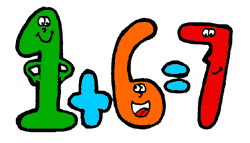Problems
327 - Subtraction Sum Again

Subtraction Sum Again

Time Limit: 1 sec

The Problem

Make a simple program that reads four variables named mn, o, p. Calculate and print the sum of subtraction of mn and subtraction of op.The Input

The input file contain a series of line. Each line contains 4 integer numbers m, n, o, and p (m<1000, n<1000, o<1000 and p<1000). Input is terminated by EOF.

The Output

For each line of input print the SUM of m - n and o - p in a separate line.

Sample Input

10 4 5 2

Sample Output

9

Problem Setter: Nezam Uddin